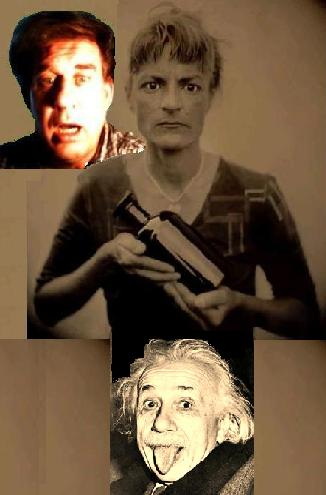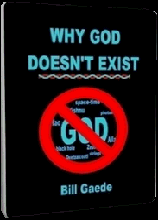The number of numbers definition of Mathematics is an a posteriori definition. The mathematician counts the
number of numbers or coordinates and proves retroactively what he meant by dimension. This results in
inconsistent conclusions. For example, if we count 400 parameters for a 3-D object, the object has 400
dimensions. No problem. But, this gives the mathematician no reason to claim that you cannot visualize the
object. The definition of Mathematics should not preclude you from seeing the object. According to
Mathematics, if you heat a cube at 3:00 p.m., the cube is now 5-D: length, width, height, 30ºC, and 3:00 p.m.
So why can’t you visualize this cube? What did the mathematical dimensions add to or subtract from the
structure of the cube that would suddenly make it invisible or unimaginable to you? Clearly, the idiots of
Mathematics are comparing apples and oranges. They are invoking the mathematical and ‘physical’
dimensions, and giving their explanation in terms of ordinary speech.

The 'number of numbers' is not a definition, but a proof. The mathematician is compelled to count the number
of coordinates needed to specify a point on a cube in order to 'conclude' that this geometric figure is 3D.

It is the proof nature of such 'definition' that leads Hawking to infer that the universe is four-dimensional
because he can specify the location of a point within it with four numbers -- one temporal and three spatial
'coordinates'. Following Hawking's logic, if we decide to specify the location of a point on a cube with four
coordinates or numbers this also makes the cube four-dimensional (Fig. 1). Indeed, orthogonality is an
integral part of the definition of a physical coordinate system.   Hence, relativists need only 'prove' that
time runs perpendicular to latitude or width if they want to insist on their definition of dimension.

Nevertheless, the mathematicians are not talking about the number of numbers, but about length, width,
and height, which they promised not to do:

“ In geometry, the tesseract is the 4-dimensional analog of the cube. That is, the tesseract
is to the cube as the cube is to the square... This structure is not easily imagined but it is
possible to project tesseracts into three- or two-dimensional spaces. Furthermore,
projections on the 2D-plane become more instructive by rearranging the positions of the
projected vertices. In this fashion, one can obtain pictures that no longer reflect the spatial
relationships within the tesseract, but which illustrate the connection structure of the
vertices” 

In physics, spacetime is a model that combines three-dimensional space and one-
dimensional time into a single construct called the space-time continuum, in which
time plays the role of the 4th dimension. According to Euclidean space perception,
our universe has three dimensions of space, and one dimension of time. By combining
the two concepts into a single manifold, physicists are able to significantly simplify the
form of most physical laws, as well as describe the workings of the universe at both
supergalactic and subatomic levels in a more uniform way.”  

Clearly, we're not talking about number of numbers needed to locate a point but about geometric structural
features.The 'number of numbers' dimension of Math is not a definition, but a proof
 Adapted for the Internet from:Why God Doesn't ExistThis one's empty too, Bill! The lousy bum! He's gotta be in the 26th dimension by now!
 ...and the 25th dimension, hic, is the coordinate of mass... and the, hic, 26th coordinate is the dimension of beer. And they're all rolled up into, hic, teensy weensy curly cues.

Pages in this module:

3.    This page:   The 'number of numbers' dimension of Math is not a definition, but a proof________________________________________________________________________________________